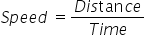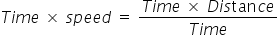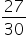Mathematics
Easy

Question

# A train travels with a constant speed of 54 miles per hour. Distance travelled by the train in 30 minutes would be ____________.

## 25313527Hint:

## The correct answer is: 27

### The given values of speed and time are as follows Speed = 54 miles per hour Time = 30 minutes We have to find the distance travelled. We know the formula for speed.e will rearrange the above formula for distance, as we don’t have it’s valueWe will multiply the L.H.S and R.H.S of the formula by time. .Time × speed = Distance.Rearranging we get.         Distance = Speed × TimeWe will substitute the values of speed and time in above equationBefore that, we will have to make the units same. So we will find the speed in miles per minute. The sleep is in miles per hour. So to convert it into miles per minute we will have to convert the hour into minutes. 1 hour means 60 minutes. So we will divide the speed by 60 minutes to get the value.We will substitute this value in the equation for distanceWe getDistance = Speed × Time.=× 30= 27 milesTherefore, the distance travelled is 27 miles.So the option which is '27’, is the right option.

We should be familiar with different units. To see if the ratio is right we can cross check the units of the quantities.

### Related Questions to study#### With Turito Foundation.#### Get an Expert Advice From Turito.